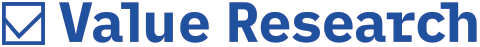FIFO Method

The FIFO method is used to calculate capital gains

I have invested in a single equity fund many times. I want to know that if I redeem some of the units now, which units will be considered as redeemed and how will the capital gain be calculated? Will the FIFO method be used for calculating the capital gains? Please clarify.
—Ajay Chandna

Yes, First In First Out (FIFO) method will be used for calculation of capital gains on the redeemed units. When you redeem your investment, the units which are bought first would be assumed to be redeemed first, then the units bought in the second lot and thereafter. The capital gains would be calculated on the above basis.